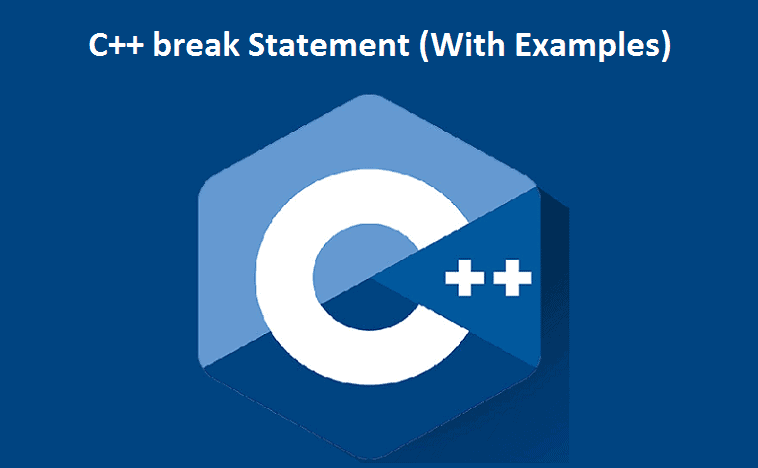# C++ break StatementC++ break Statement: In this tutorial, we will find out about the break statement and its working in loops with the help of examples.

In computer programming, the break statement is used to terminate the loop in which it is used.

The syntax of the break statement is:

``break;``

Before you learn about the break statement, make sure you know about:

C++ for loop

C++ if…else

C++ while loop

Contents

## Example 1: break with for loop

``````// program to print the value of i

#include <iostream>
using namespace std;

int main() {
for (int i = 1; i <= 5; i++) {
// break condition
if (i == 3) {
break;
}
cout << i << endl;
}

return 0;
}``````

Output

``````1
2``````

In the above program, the for loop is used to print the value of i in each iteration. Here, notice the code:

``````if (i == 3) {
break;
}``````

This implies, when i is equivalent to 3, the break statement ends the loop. Hence, the output doesn’t include values greater than or equal to 3.

Note: The break statement is normally used with decision-making statements.

## Example 2: break with while loop

``````// program to find the sum of positive numbers
// if the user enters a negative numbers, break ends the loop
// the negative number entered is not added to sum

#include <iostream>
using namespace std;

int main() {
int number;
int sum = 0;

while (true) {
// take input from the user
cout << "Enter a number: ";
cin >> number;

// break condition
if (number < 0) {
break;
}

sum += number;
}

// display the sum
cout << "The sum is " << sum << endl;

return 0;
}``````

Output

``````Enter a number: 1
Enter a number: 2
Enter a number: 3
Enter a number: -5
The sum is 6. ``````

In the above program, the user enters a number. The while loop is used to print the complete whole of numbers entered by the user. Here, notice the code,

``````if(number < 0) {
break;
}``````

This means, when the user enters a negative number, the break statement terminates the loop and codes outside the loop are executed.

The while loop continues until the user enters a negative number.

## break with Nested loop

When the break is used with nested loops, break terminates the inner loop. For example,

``````// using break statement inside
// nested for loop

#include <iostream>
using namespace std;

int main() {
int number;
int sum = 0;

// nested for loops

// first loop
for (int i = 1; i <= 3; i++) {
// second loop
for (int j = 1; j <= 3; j++) {
if (i == 2) {
break;
}
cout << "i = " << i << ", j = " << j << endl;
}
}

return 0;
}
``````

Output

``````i = 1, j = 1
i = 1, j = 2
i = 1, j = 3
i = 3, j = 1
i = 3, j = 2
i = 3, j = 3``````

In the above program, the break statement is executed when I == 2. It ends the inner loop, and the control stream of the program moves to the external loop.

Hence, the value of I = 2 is never shown in the output.

Please feel free to give your comment if you face any difficulty here.### 18 of the Best Hacking Apps on Android### 5 of the Best Android Apps to Back Up Your Phone’s Data